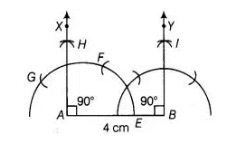# Draw a line segment AB of 4 cm in length.`
Question:

Draw a line segment AB of 4 cm in length. Draw a line perpendicular to AB through A and B, respectively. Are these lines parallel?

Solution:

To draw a line perpendicular to AB through A and B, respectively. Use the following steps of construction.

1. Draw a line segment AB = 4 cm.
2. Taking 4 as centre and radius more than ½ AB (i.e., 2 cm) draw an arc say it intersect AB at E.
3. Taking E as centre and with same radius as above draw an arc which intersect previous arc at F.
4.  Again, taking F as centre and with same radius as above draw an arc which intersect previous arc (obtained in step ii) at G.1. Taking G and F are centres, draw arcs which intersect each other at H.
2. Join AH . Thus, AX is perpendicular to AB at A. Similarly, draw BY ⊥ AB at B.
Now, we know that if two lines are parallel, then the angle between them will be 0° or
180°.
Here, ∠XAB = 90° [∴ XA ⊥ AB]
and ∠YBA = 90° [ ∴ YB ⊥ AB]
∠XAB+ ∠YBA = 90° + 90° = 180°
So, the lines XA and YS are parallel.
[since, it sum of interior angle on same side of transversal is 180°, then the two lines are
parallel]